# ChartTypes

5 Feb 201824 minutes to read

## Line Chart

To render a line chart, set the series type to Line in the chart series. To change the line segment color, use the Fill property of the series.

• CSHTML
• ``````<ej-chart id="chartContainer">
// ...
<e-chart-series>
<e-series type="Line" fill="#E94649"></e-series>
</e-chart-series>
// ...
</ej-chart>``````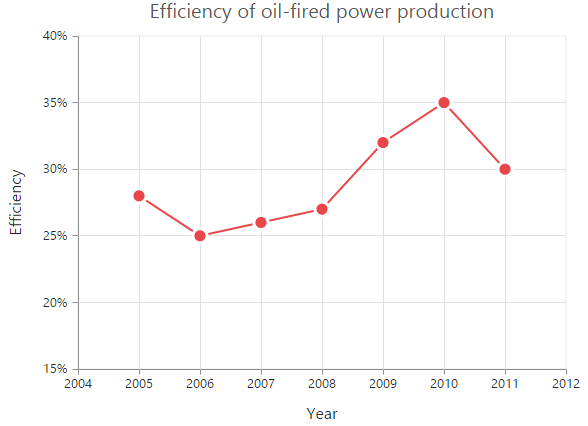### Change the line width

To change the width of the line segment, use the Width property of the series.

• CSHTML
• ``````<ej-chart id="chartContainer">
// ...
<e-chart-series>
<e-series width="3"></e-series>
</e-chart-series>
// ...
</ej-chart>``````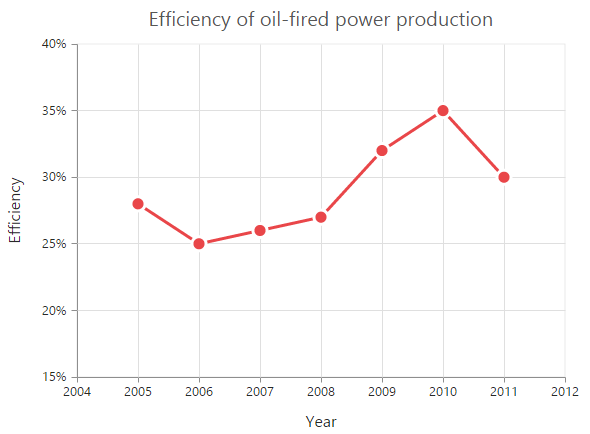### Dashed lines

To render a line chart with dotted lines, use the DashArray option of the series.

• CSHTML
• ``````<ej-chart id="chartContainer">
// ...
<e-chart-series>
<e-series dash-array="5,5"></e-series>
</e-chart-series>
// ...
</ej-chart>``````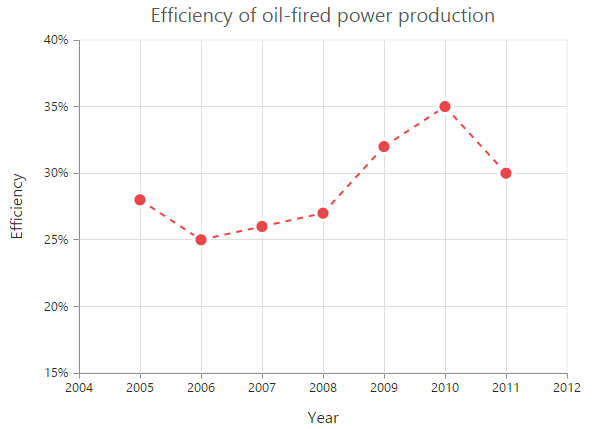### Changing the line cap

For customizing the start and end caps of the line segment, use the LineCap property.

• CSHTML
• ``````<ej-chart id="chartContainer">
// ...
<e-chart-series>
<e-series line-cap="Square"></e-series>
</e-chart-series>
// ...
</ej-chart>``````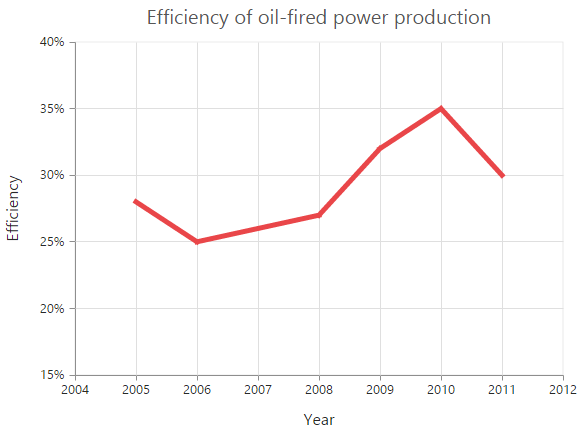### Changing the line join

To specify how the two intersecting line segments should be joined, use the LineJoin property.

• CSHTML
• ``````<ej-chart id="chartContainer">
// ...
<e-chart-series>
<e-series line-join="Round"></e-series>
</e-chart-series>
// ...
</ej-chart>``````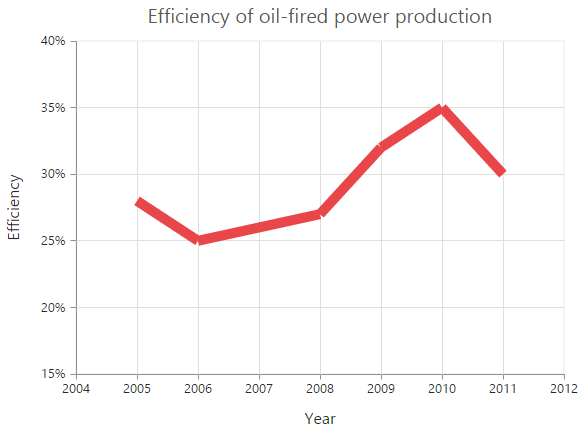### Multi color line

You can change the color of the line segments by using the Fill property of the each Points in the series.

• CSHTML
• ``````<ej-chart id="chartContainer">
// ...
<e-chart-series>
<e-series>
<e-points><e-point fill="red"></e-point></e-points>
</e-series>
</e-chart-series>
// ...
</ej-chart>``````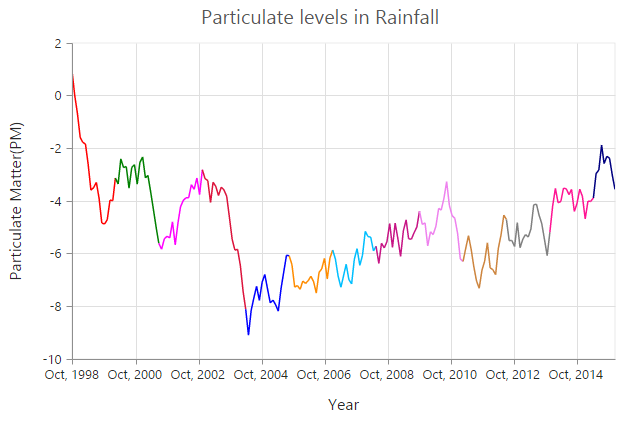## Step Line Chart

To render a step line chart, set the series type to StepLine” in the chart series. To change the StepLine’s segment color, use the Fill property of the series.

• CSHTML
• ``````<ej-chart id="chartContainer">
// ...
<e-chart-series>
<e-series type="StepLine" fill="#E94649">
</e-series>
</e-chart-series>
// ...
</ej-chart>``````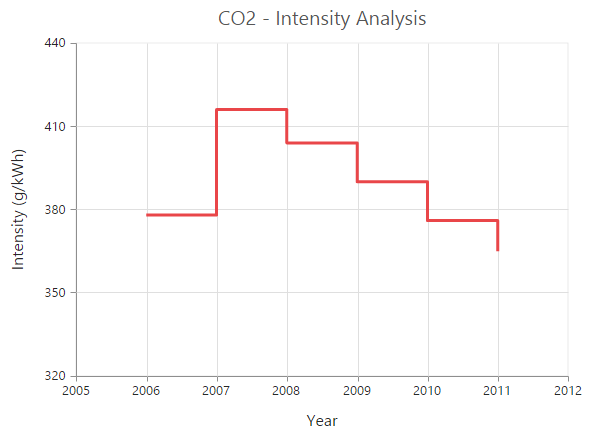### Changing the line width

To change the line width, use the Width property.

• CSHTML
• ``````<ej-chart id="chartContainer">
// ...
<e-chart-series>
<e-series width="3"></e-series>
</e-chart-series>
// ...
</ej-chart>``````### Dashed lines

To render the step line series with dotted lines, use the DashArray option of the series.

• CSHTML
• ``````<ej-chart id="chartContainer">
// ...
<e-chart-series>
<e-series dash-array="5,5"></e-series>
</e-chart-series>
// ...
</ej-chart>``````### Changing the line cap

For customizing the start and end caps of the line segment, use the LineCap property.

• CSHTML
• ``````<ej-chart id="chartContainer">
// ...
<e-chart-series>
<e-series line-cap="Square"></e-series>
</e-chart-series>
// ...
</ej-chart>``````### Changing the line join

To specify how the two intersecting line segments should be joined, use the LineJoin property.

• CSHTML
• ``````<ej-chart id="chartContainer">
// ...
<e-chart-series>
<e-series line-join="Round"></e-series>
</e-chart-series>
// ...
</ej-chart>``````## Area Chart

To render an area chart, specify the series type to Area in the chart series. To change the area color, use the Fill property of the series.

• CSHTML
• ``````<ej-chart id="chartContainer">
<e-chart-series>
<e-series type="Area" fill="#69D2E7">
</e-series>
</e-chart-series>
</ej-chart>``````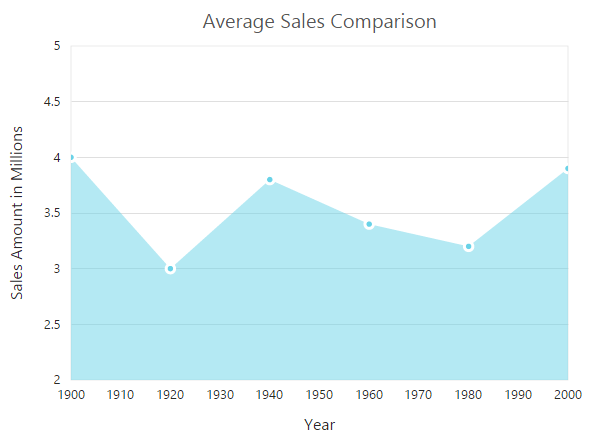## Range Area Chart

To render a range area chart, set the type to RangeArea in the chart series. To change the range area color, use the Fill property of the series.

Since the range area series requires two y values for a point, add High and Low values. High and Low values specify the maximum and minimum ranges of the points.

• When you are using the Points option, specify the high and low values by using the High and Low option of the point.

• When you are using the DataSource option to assign the data, map the fields from the data source that contains high and low values by using the series.High and Series.Low options.

• CSHTML
• ``````<ej-chart id="chartContainer">
<e-chart-series>
<e-series type="RangeArea" fill="Indigo">
<e-points><e-point x="1935" high="80" low="70"></e-point></e-points>
</e-series>
</e-chart-series>
</ej-chart>``````## Step Area Chart

To render a step area chart, set the type to StepArea in the chart series. To change the step area color, use the Fill property of the series.

• CSHTML
• ``````<ej-chart id="chartContainer">
// ...
<e-chart-series>
<e-series type="StepArea" fill="#69D2E7"></e-series>
</e-chart-series>
// ...
</ej-chart>``````## Spline Area Chart

To render a spline area chart, set the type to SplineArea in the chart series. To change the spline area color, use the Fill property of the series.

• CSHTML
• ``````<ej-chart id="chartContainer">
// ...
<e-chart-series>
<e-series type="SplineArea" fill="#C4C24A"></e-series>
</e-chart-series>
// ...
</ej-chart>``````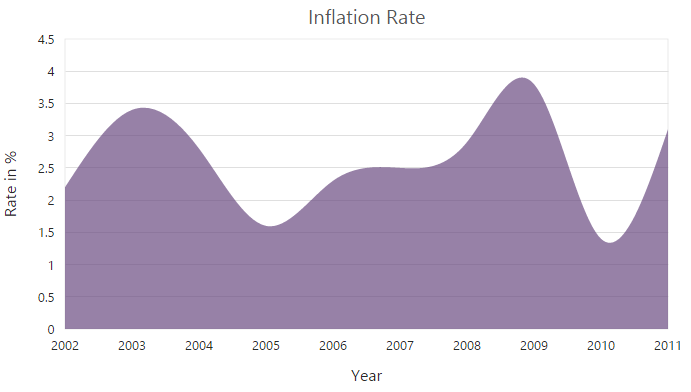## Stacked Area Chart

To render a stacked area chart, set the type to StackingArea in the chart series. To change the stacking area color, use the Fill property of the series.

• CSHTML
• ``````<ej-chart id="chartContainer">
// ...
<e-chart-series>
<e-series type="StackingArea" fill="#69D2E7"></e-series>
</e-chart-series>
// ...
</ej-chart>``````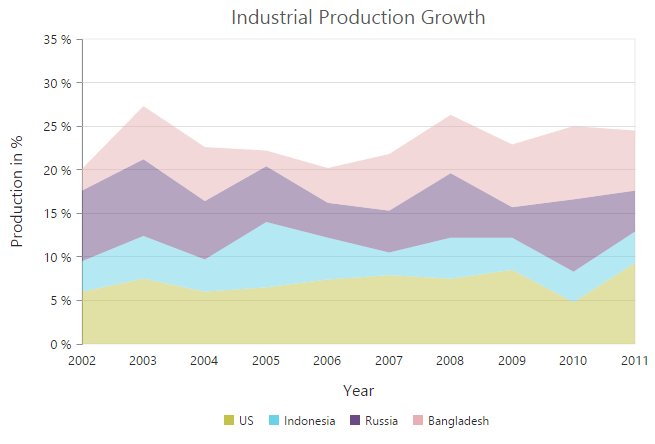## 100% Stacked Area Chart

To render a 100% stacked area chart, set the type to StackingArea100 in the chart series. To change the StackingArea100 color, use the Fill property of the series.

• CSHTML
• ``````<ej-chart id="chartContainer">
// ...
<e-chart-series>
<e-series type="StackingArea100" fill="#C4C24A"></e-series>
</e-chart-series>
// ...
</ej-chart>``````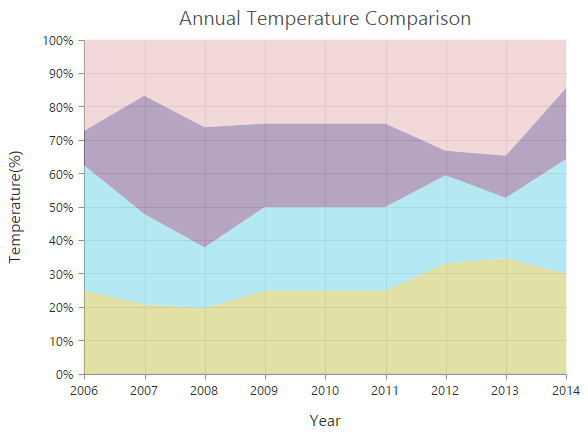## Stacked Spline Area Chart

To render a stacked spline area chart, set the type to StackingSplineArea in the chart series.

• CSHTML
• ``````<ej-chart id="chartContainer">
// ...
<e-chart-series>
<e-series type="StackingSplineArea"></e-series>
</e-chart-series>
// ...
</ej-chart>``````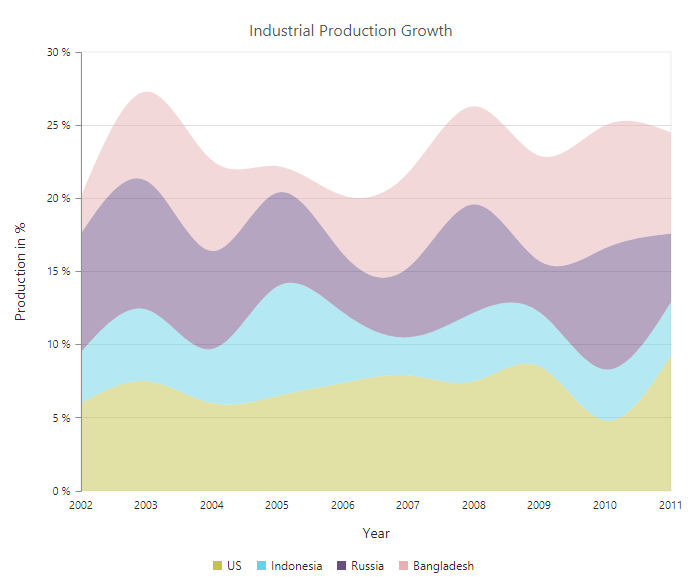## 100% Stacked Spline Area Chart

To render a 100% stacked spline area chart, set the type to StackingSplineArea100 in the chart series.

• CSHTML
• ``````<ej-chart id="chartContainer">
// ...
<e-chart-series>
<e-series type="StackingSplineArea100"></e-series>
</e-chart-series>
// ...
</ej-chart>``````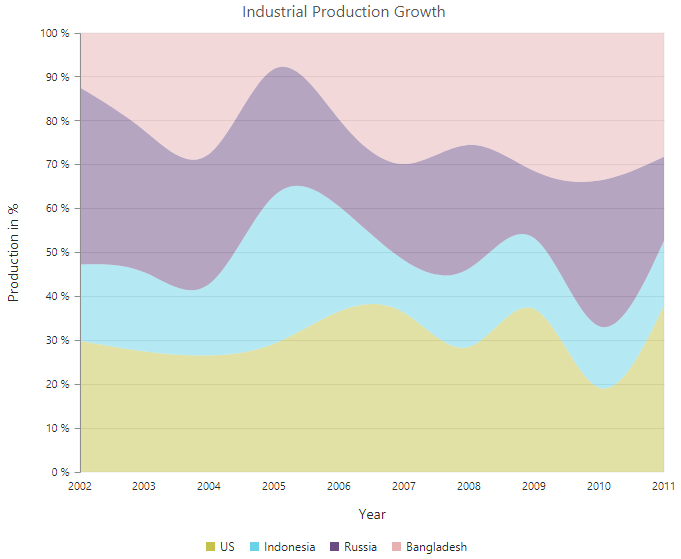## Column Chart

To render a column chart, set the type to Column in the chart series. To change the color of the column series, use the Fill property.

• CSHTML
• ``````<ej-chart id="chartContainer">
// ...
<e-chart-series>
<e-series type="Column" fill="#E94649"></e-series>
</e-chart-series>
// ...
</ej-chart>``````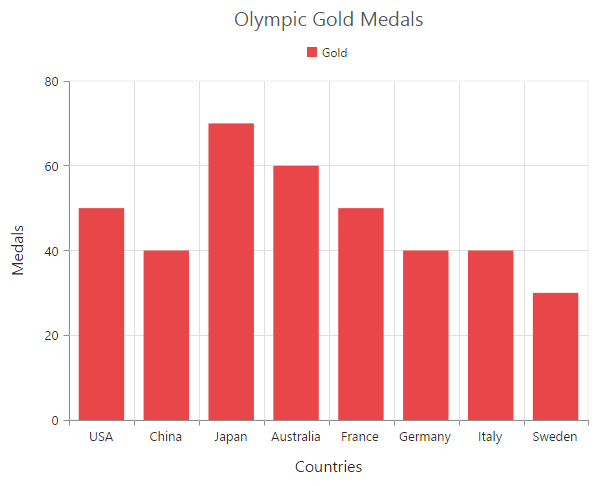### Change a point color

You can change the color of a column by using the Fill property of the point.

• CSHTML
• ``````<ej-chart id="chartContainer">
// ...
<e-chart-series>
<e-series>
<e-points><e-point fill="skyblue"></e-point></e-points>
</e-series>
</e-chart-series>
// ...
</ej-chart>``````### Column width customization

Width of any column type series can be customized by using the ColumnWidth property. Default value of ColumnWidth is 0.7. This value ranges from 0 to 1. Here 1 corresponds to 100% of available width and 0 corresponds to 0% of available width.

NOTE

Width of a column also depends on the ColumnSpacing property, because ColumnSpacing will reduce the space available for drawing a column. This is also applicable for stacking column, stacking column 100, bar, stacking bar, stacking bar 100, range column, HiLo, HiLo open close, candle, and waterfall charts.

• CSHTML
• ``````<ej-chart id="chartContainer">
// ...
<e-chart-series>
<e-series column-width="0.8">
</e-series>
</e-chart-series>
// ...
</ej-chart>``````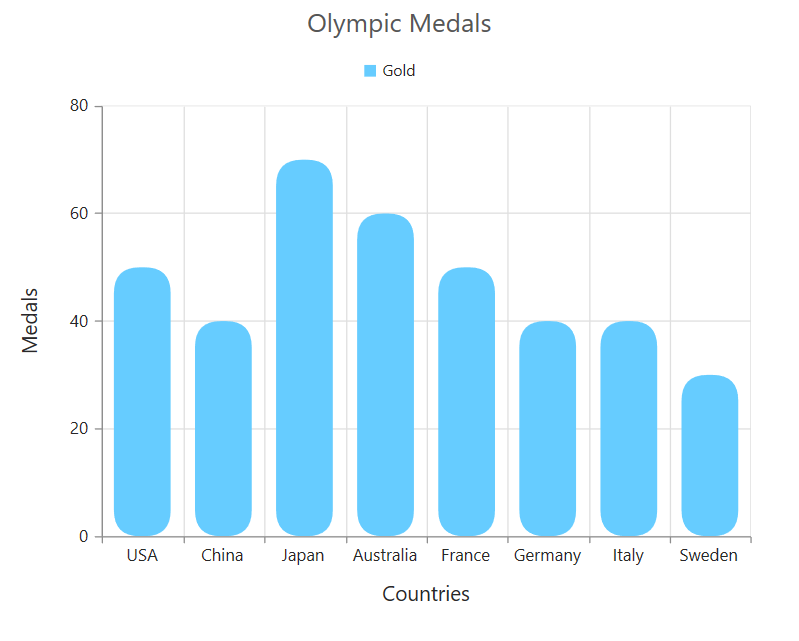### Column with rounded corners

Corners of the column chart can be customized by setting value to the CornerRadius property.

• CSHTML
• ``````<ej-chart id="chartContainer">
// ...
// ...
</ej-chart>``````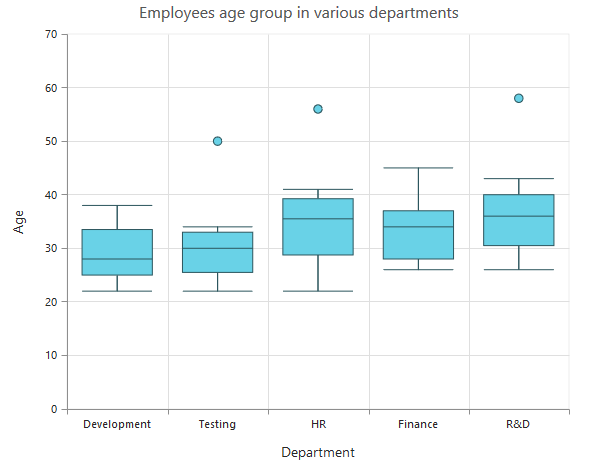### Spacing between column series

Spacing between column type series can be customized by using the ColumnSpacing property. Default value of ColumnSpacing is 0. This value ranges from 0 to 1. Here 1 corresponds to 100% available space and 0 corresponds to 0% available space.

NOTE

Space between the columns will also affect the width of the column. For example, setting 20% spacing and 100% width will render columns with 80% of total width. This is also applicable for stacking column, stacking column 100, bar, stacking bar, stacking bar 100, range column, HiLo, HiLo open close, candle, and waterfall charts.

• CSHTML
• ``````<ej-chart id="chartContainer">
// ...
<e-chart-series>
<e-series column-spacing="0.2">
</e-series>
</e-chart-series>
// ...
</ej-chart>``````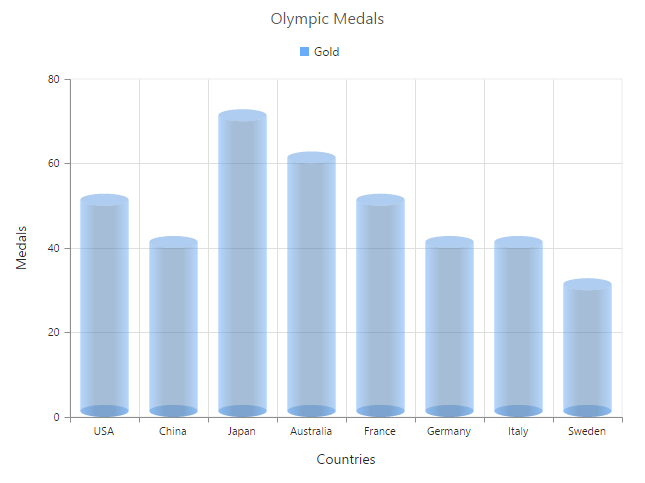### Cylindrical Chart

To render a cylindrical chart, set the ColumnFacet property to “Cylinder” in the chart series along with the series type.

The following chart types can be rendered as cylinder in both 2D and 3D views:

• Column chart, bar chart, stacked column chart, stacked bar chart, 100% stacked column chart, 100% stacked bar chart.
• CSHTML
• ``````<ej-chart id="chartContainer">
// ...
<e-chart-series>
<e-series type="Line" column-facet="Cylinder">
</e-series>
</e-chart-series>
// ...
</ej-chart>``````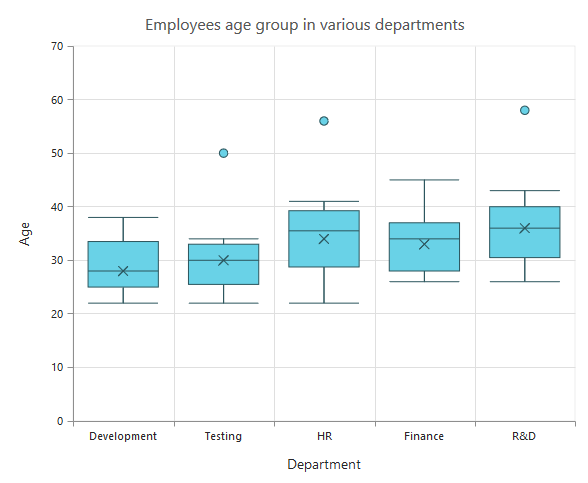## Range Column Chart

To render a range column chart, set the type to RangeColumn in the chart series. To change the range column color, use the Fill property of the series.

Since the range column series requires two y values for a point, add the High and Low values. High and Low values specify the maximum and minimum ranges of the points.

• When you are using the Points option, specify the high and low values by using the High and Low options of the point.

• When you are using the DataSource option to assign the data, map the fields from the datasource that contains high and low values by using the Series.High and Series.Low options.

• CSHTML
• ``````<ej-chart id="chartContainer">
// ...
<e-chart-series>
<e-series type="RangeColumn" fill="#E94649">
<e-points><e-point high="6.1" low="0.7"></e-point></e-points>
</e-series>
</e-chart-series>
// ...
</ej-chart>``````### Change a point color

To change the color of a range column, use the Fill property of point.

• CSHTML
• ``````<ej-chart id="chartContainer">
// ...
<e-chart-series>
<e-series>
<e-points><e-point fill="skyblue"></e-point></e-points>
</e-series>
</e-chart-series>
// ...
</ej-chart>``````## Stacked Column Chart

To render a stacked column chart, set the type to StackingColumn in the chart series. To change the stacking column color, use the Fill property of the series.

• CSHTML
• ``````<ej-chart id="chartContainer">
// ...
<e-chart-series>
<e-series type="StackingColumn" fill="#E94649">
</e-series>
</e-chart-series>
// ...
</ej-chart>``````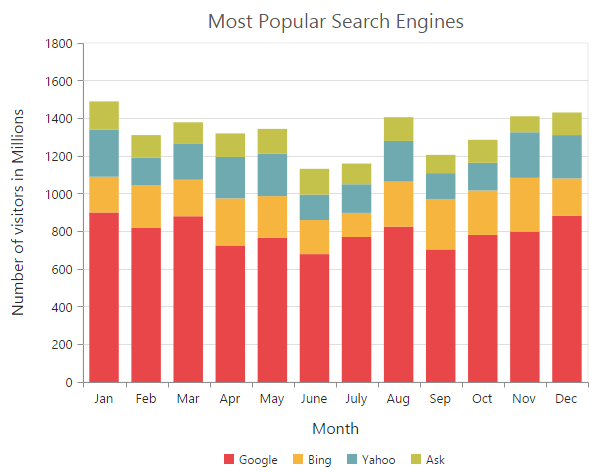### Cluster/Group stacked columns

You can use the StackingGroup property to group the stacked columns. Columns with same group name are stacked on top of each other.

• CSHTML
• ``````<ej-chart id="chartContainer">
// ...
<e-chart-series>
// For grouping stacked columns
<e-series stacking-group="GroupOne">
</e-series>
// For grouping stacked columns
<e-series stacking-group="GroupOne">
</e-series>
</e-chart-series>
// ...
</ej-chart>``````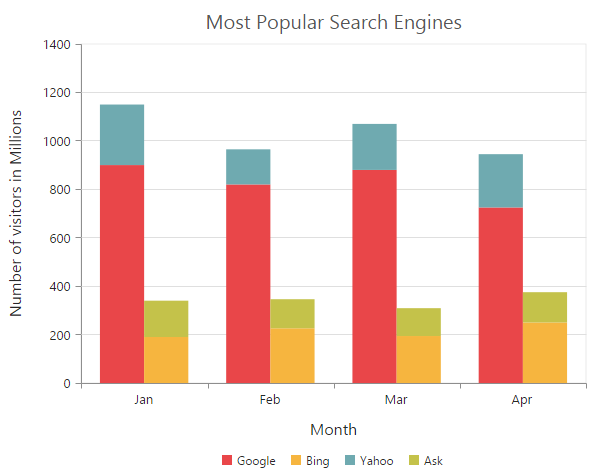### Change a point color

To change the color of a stacking column, use the Fill property of the point.

• CSHTML
• ``````<ej-chart id="chartContainer">
// ...
<e-chart-series>
<e-series>
<e-points><e-point fill="skyblue"></e-point></e-points>
</e-series>
</e-chart-series>
// ...
</ej-chart>``````## 100% Stacked Column Chart

To render a 100% stacked column chart, set the type to StackingColumn100 in the chart series. To change the stacking column 100 color, use the Fill property of the series.

• CSHTML
• ``````<ej-chart id="chartContainer">
// ...
<e-chart-series>
<e-series type="StackingColumn100" fill="#E94649">
</e-series>
</e-chart-series>
// ...
</ej-chart>``````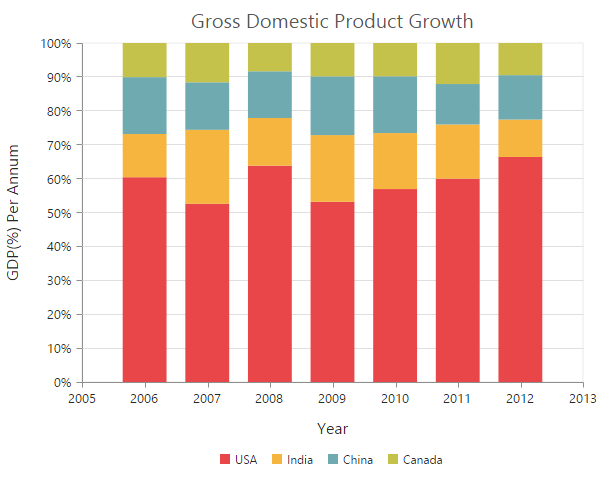### Cluster/Group 100% stacked columns

By using the StackingGroup property, you can group the 100% stacking columns. Columns with same group name are stacked on top of each other.

• CSHTML
• ``````<ej-chart id="chartContainer">
// ...
<e-chart-series>
// For grouping stacked columns
<e-series stacking-group="GroupOne">
</e-series>
// For grouping stacked columns
<e-series stacking-group="GroupOne">
</e-series>
</e-chart-series>
// ...
</ej-chart>``````### Change a point color

To change the color of a 100% stacking column, use the Fill property of the point.

• CSHTML
• ``````<ej-chart id="chartContainer">
// ...
<e-chart-series>
<e-series>
<e-points><e-point fill="skyblue"></e-point></e-points>
</e-series>
</e-chart-series>
// ...
</ej-chart>``````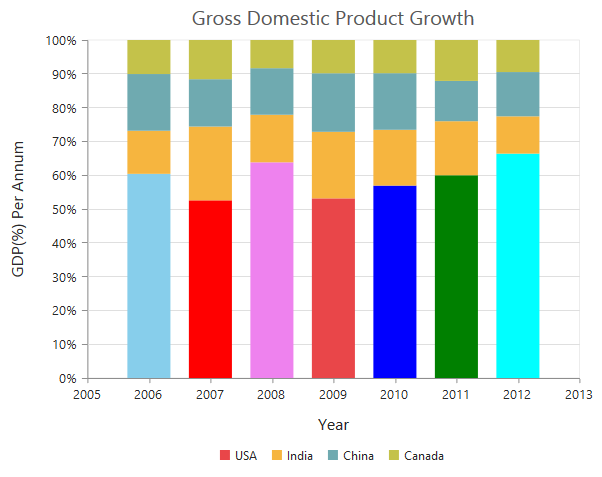## Bar Chart

To render a bar Chart, set the type to Bar in the chart series. To change the bar color, use the Fill property of the series.

• CSHTML
• ``````<ej-chart id="chartContainer">
// ...
<e-chart-series>
<e-series type="Bar" fill="#E94649">
</e-series>
</e-chart-series>
// ...
</ej-chart>``````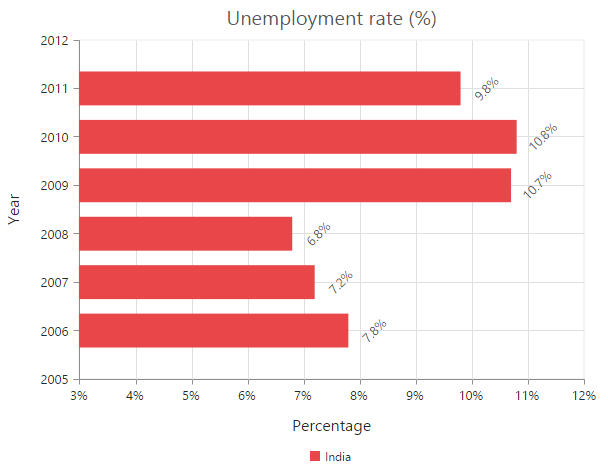### Change the color of a bar

By using the Fill property of the point, you can change the specific point of the series.

• CSHTML
• ``````<ej-chart id="chartContainer">
// ...
<e-chart-series>
<e-series>
<e-points><e-point fill="skyblue"></e-point></e-points>
</e-series>
</e-chart-series>
// ...
</ej-chart>``````## Stacked Bar Chart

To render a stacked bar chart, set the type to StackingBar in the chart series. To change the stacking bar color, use the Fill property of the series.

• CSHTML
• ``````<ej-chart id="chartContainer">
// ...
<e-chart-series>
<e-series type="StackingBar" fill="#E94649">
</e-series>
</e-chart-series>
// ...
</ej-chart>``````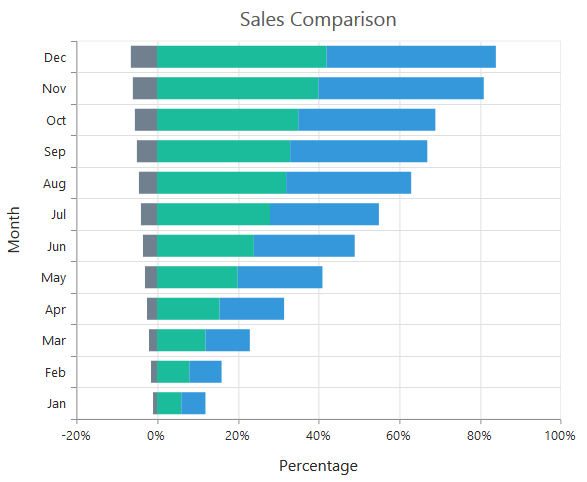### Cluster/Group stacked bars

You can use the StackingGroup property to group the stacking bars with the same group name.

• CSHTML
• ``````<ej-chart id="chartContainer">
// ...
<e-chart-series>
// For grouping stacked columns
<e-series stacking-group="GroupOne">
</e-series>
// For grouping stacked columns
<e-series stacking-group="GroupOne">
</e-series>
</e-chart-series>
// ...
</ej-chart>``````### Change a point color

You can change the color of a stacking bar by using the Fill property of the point.

• CSHTML
• ``````<ej-chart id="chartContainer">
// ...
<e-chart-series>
<e-series>
<e-points><e-point fill="skyblue"></e-point></e-points>
</e-series>
</e-chart-series>
// ...
</ej-chart>``````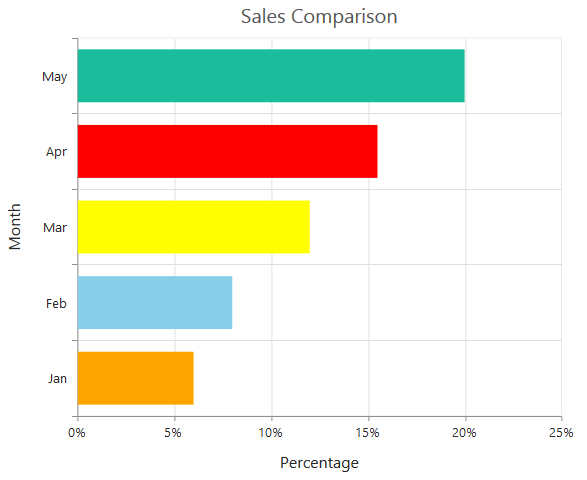## 100% Stacked Bar Chart

To render a 100% stacked bar chart, set the type to StackingBar100 in the chart series. To change the stacking bar 100 color, use the Fill property of the series.

• CSHTML
• ``````<ej-chart id="chartContainer">
// ...
<e-chart-series>
<e-series type="StackingBar100" fill="#E94649">
</e-series>
</e-chart-series>
// ...
</ej-chart>``````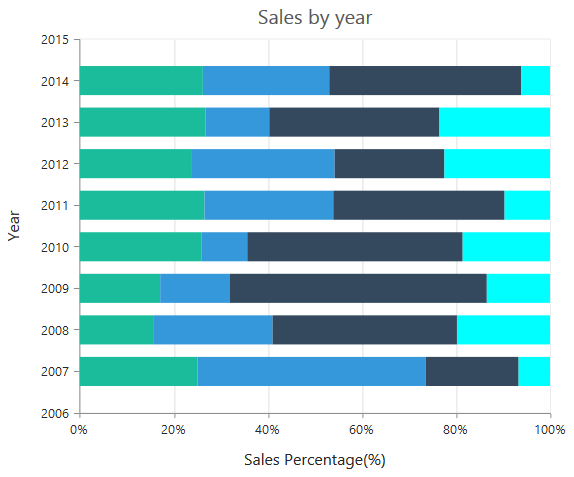By using the StackingGroup property, you can group the 100% stacking bars with the same group name.

• CSHTML
• ``````<ej-chart id="chartContainer">
// ...
<e-chart-series>
// For grouping stacked columns
<e-series stacking-group="GroupOne">
</e-series>
// For grouping stacked columns
<e-series stacking-group="GroupOne">
</e-series>
</e-chart-series>
// ...
</ej-chart>``````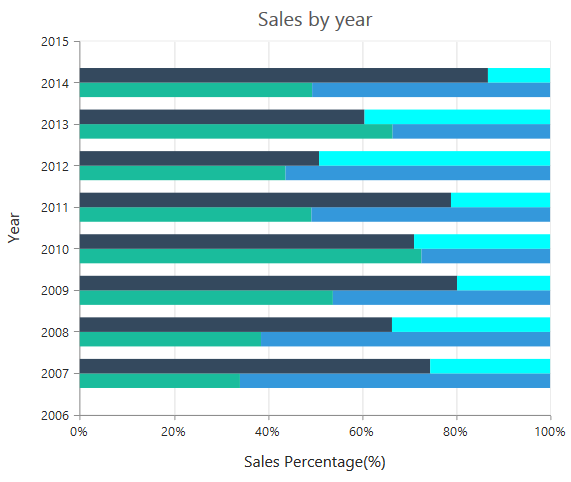### Change a point color

To change the color of a 100% stacking bar, use the Fill property of the point.

• CSHTML
• ``````<ej-chart id="chartContainer">
// ...
<e-chart-series>
<e-series>
<e-points><e-point fill="skyblue"></e-point></e-points>
</e-series>
</e-chart-series>
// ...
</ej-chart>``````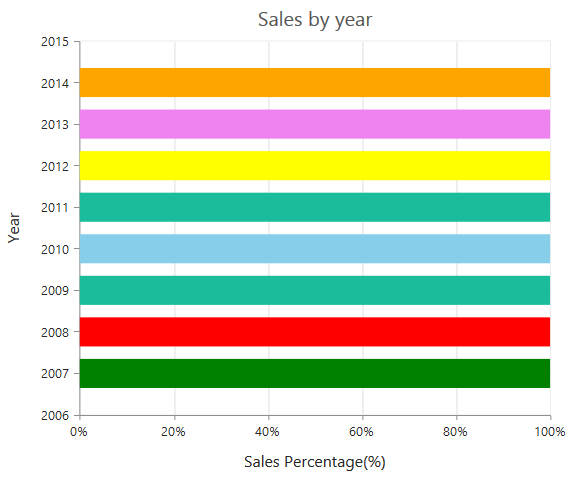## Spline Chart

To render a spline chart, set the type to Spline in the chart series. To change the spline segment color, use the Fill property of the series.

• CSHTML
• ``````<ej-chart id="chartContainer">
// ...
<e-chart-series>
<e-series type="Spline" fill="#E94649">
</e-series>
</e-chart-series>
// ...
</ej-chart>``````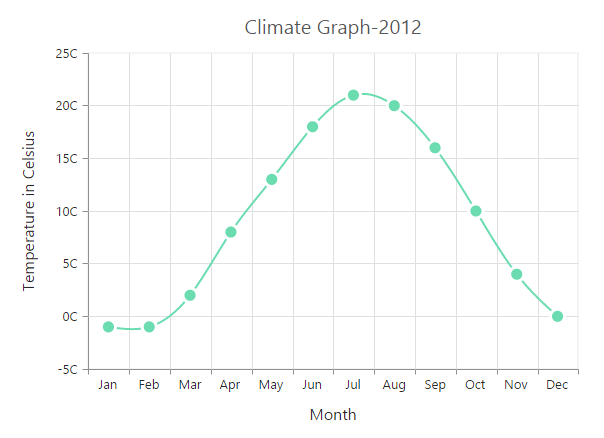### Spline types

Spline series supports four types of curves, namely natural, monotonic, cardinal, and clamped. To change the spline type, use the SplineType property in the series.

• CSHTML
• ``````<ej-chart id="chartContainer">
// ...
<e-chart-series>
<e-series type="Spline" spline-type="Natural">
</e-series>
</e-chart-series>
// ...
</ej-chart>``````

### Change the cardinal spline tension

To change cardinal spline tension, use the `cardinalSplineTension` property of the series. The default value of cardinalSplineTension is 0.5. This value ranges from 0 to 1.

• CSHTML
• ``````<ej-chart id="chartContainer">
// ...
<e-chart-series>
<e-series type="Spline" spline-type="Cardinal" cardinal-spline-tension="0.7">
</e-series>
</e-chart-series>
// ...
</ej-chart>``````

### Change the spline width

To change the width of the spline segment, use the Width property of the series.

• CSHTML
• ``````<ej-chart id="chartContainer">
// ...
<e-chart-series>
<e-series width="3"></e-series>
</e-chart-series>
// ...
</ej-chart>``````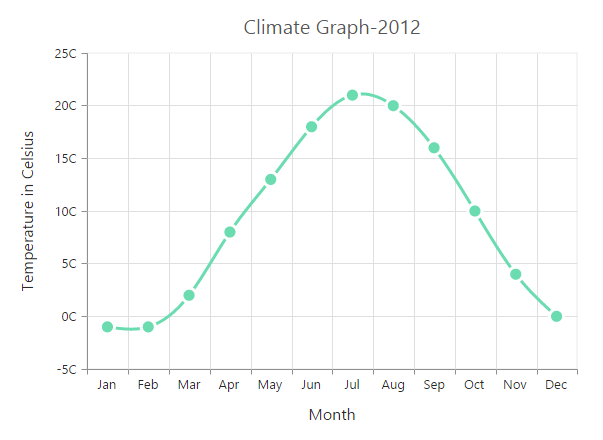### Dashed lines

To render the spline chart with dotted lines, use the DashArray option of the series.

• CSHTML
• ``````<ej-chart id="chartContainer">
// ...
<e-chart-series>
<e-series dash-array="5,5"></e-series>
</e-chart-series>
// ...
</ej-chart>``````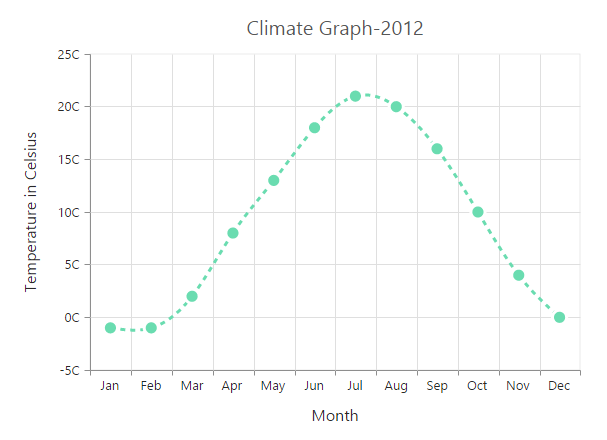## Pie Chart

You can create a pie chart by setting the series type to Pie in the chart series.

• CSHTML
• ``````<ej-chart id="chartContainer">
// ...
<e-chart-series>
<e-series type="Pie"></e-series>
</e-chart-series>
// ...
</ej-chart>``````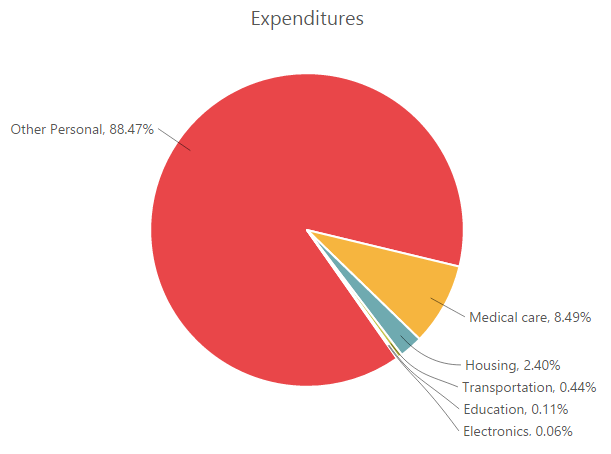### Change the pie size

You can use the PieCoefficient property to change the diameter of the pie chart with respect to the plot area. It ranges from 0 to 1, and the default value is 0.8.

• CSHTML
• ``````<ej-chart id="chartContainer">
// ...
<e-chart-series>
<e-series pie-coefficient="0.4f"></e-series>
</e-chart-series>
// ...
</ej-chart>``````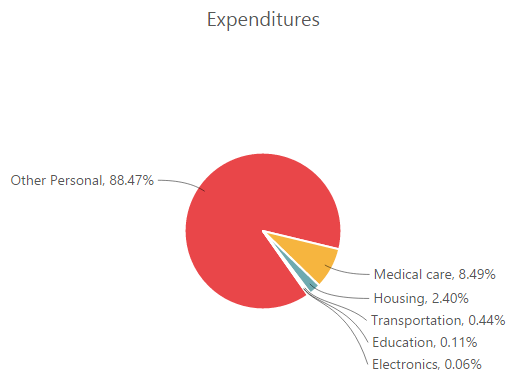### Explode a pie segment

You can explode a pie segment on the chart load by using the ExplodeIndex property of the series.

• CSHTML
• ``````<ej-chart id="chartContainer">
// ...
<e-chart-series>
<e-series explode-index="1">
</e-series>
</e-chart-series>
// ...
</ej-chart>``````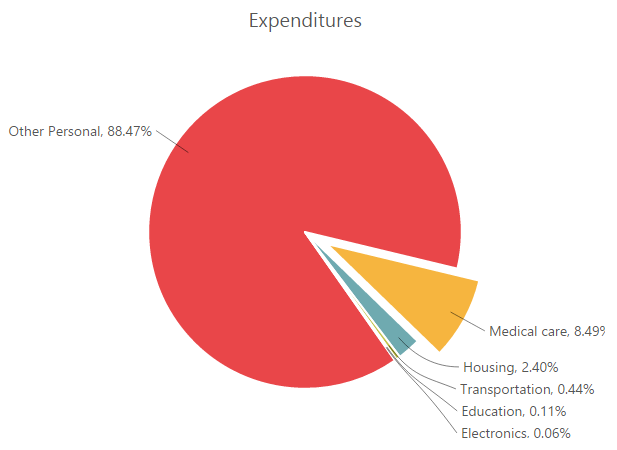### Explode all the segments

To explode all the segments of the pie chart, enable the ExplodeAll property.

• CSHTML
• ``````<ej-chart id="chartContainer">
// ...
<e-chart-series>
<e-series explode-all="true">
</e-series>
</e-chart-series>
// ...
</ej-chart>``````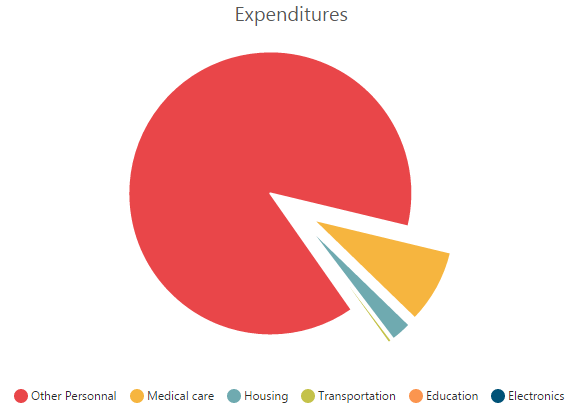### Explode a pie segment by hovering the mouse

To explode a pie segment by hovering the mouse, enable the Explode property of the series.

• CSHTML
• ``````<ej-chart id="chartContainer">
// ...
<e-chart-series>
<e-series explode="true">
</e-series>
</e-chart-series>
// ...
</ej-chart>``````### Sector of Pie

Chart allows you to render all the data points/segments in the semi-pie, quarter-pie, or in any sector by using the StartAngle and EndAngle options.

• CSHTML
• ``````<ej-chart id="chartContainer">
// ...
<e-chart-series>
<e-series type="Pie" start-angle="-90" end-angle="90">
</e-series>
</e-chart-series>
// ...
</ej-chart>``````## Doughnut Chart

To create a doughnut chart, specify the series type to Doughnut in the chart series.

• CSHTML
• ``````<ej-chart id="chartContainer">
// ...
<e-chart-series>
<e-series type="Doughnut">
</e-series>
</e-chart-series>
// ...
</ej-chart>``````You can change the doughnut chart inner radius by using the DoughnutCoefficient with respect to the plot area. It ranges from 0 to 1 and the default value is 0.4.

• CSHTML
• ``````<ej-chart id="chartContainer">
// ...
<e-chart-series>
<e-series doughnut-coefficient="0.6f">
</e-series>
</e-chart-series>
// ...
</ej-chart>``````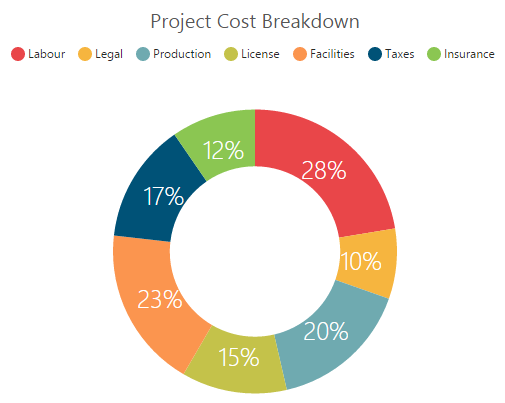### Change the doughnut size

You can use the DoughnutSize property to change the diameter of the doughnut chart with respect to the plot area. It ranges from 0 to 1, and the default value is 0.8.

• CSHTML
• ``````<ej-chart id="chartContainer">
// ...
<e-chart-series>
<e-series doughnut-size="0.4f">
</e-series>
</e-chart-series>
// ...
</ej-chart>``````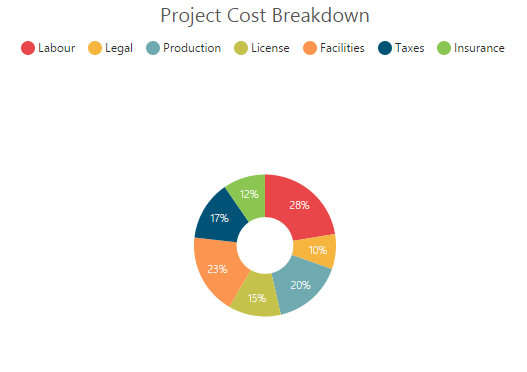### Explode a doughnut segment

To explode a specific doughnut segment, set the index to be exploded by using the ExplodeIndex property of the series.

• CSHTML
• ``````<ej-chart id="chartContainer">
// ...
<e-chart-series>
<e-series explode-index="1">
</e-series>
</e-chart-series>
// ...
</ej-chart>``````### Explode all the segments

To explode all the segments, enable the ExplodeAll property of the series.

• CSHTML
• ``````<ej-chart id="chartContainer">
// ...
<e-chart-series>
<e-series explode-all="true">
</e-series>
</e-chart-series>
// ...
</ej-chart>``````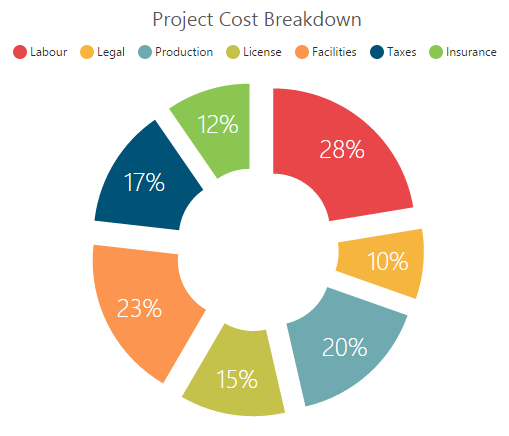### Explode a doughnut segment by hovering the mouse

To explode a doughnut segment by hovering the mouse, enable the Explode property of the series.

• CSHTML
• ``````<ej-chart id="chartContainer">
// ...
<e-chart-series>
<e-series explode="true">
</e-series>
</e-chart-series>
// ...
</ej-chart``````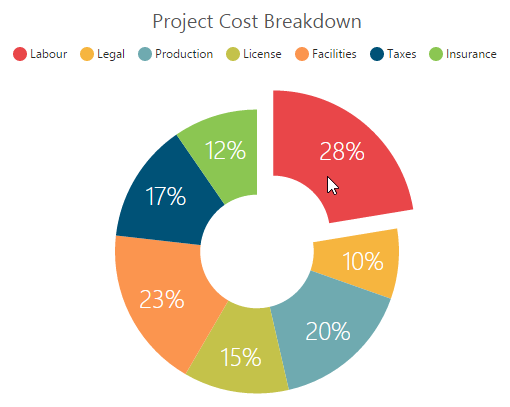### Sector of doughnut

Chart allows you to render all the data points/segments in the semi-doughnut, quarter- doughnut, or in any sector by using the StartAngle and EndAngle options.

• CSHTML
• ``````<ej-chart id="chartContainer">
// ...
<e-chart-series>
<e-series type="Doughnut" start-angle="-90" end-angle="90">
</e-series>
</e-chart-series>
// ...
</ej-chart>``````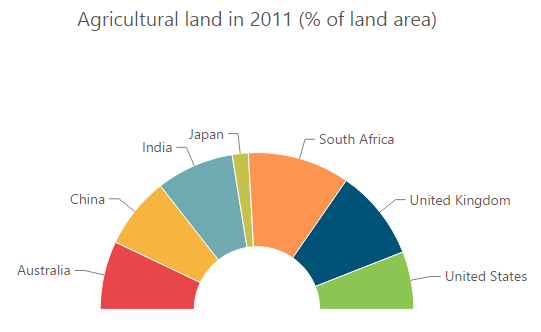## Multiple Pie Chart

Chart provides support to render more than one series in pie and doughnut chart. Radius of each series is calculated based on the radius of the previous series. In addition, legend is displayed according to the list of chart series.

• CSHTML
• ``````<ej-chart id="chartContainer">
// ...
<e-chart-series>
<e-series type="Pie">
</e-series>
<e-series type="Pie">
</e-series>
</e-chart-series>
// ...
</ej-chart>``````

Multiple pie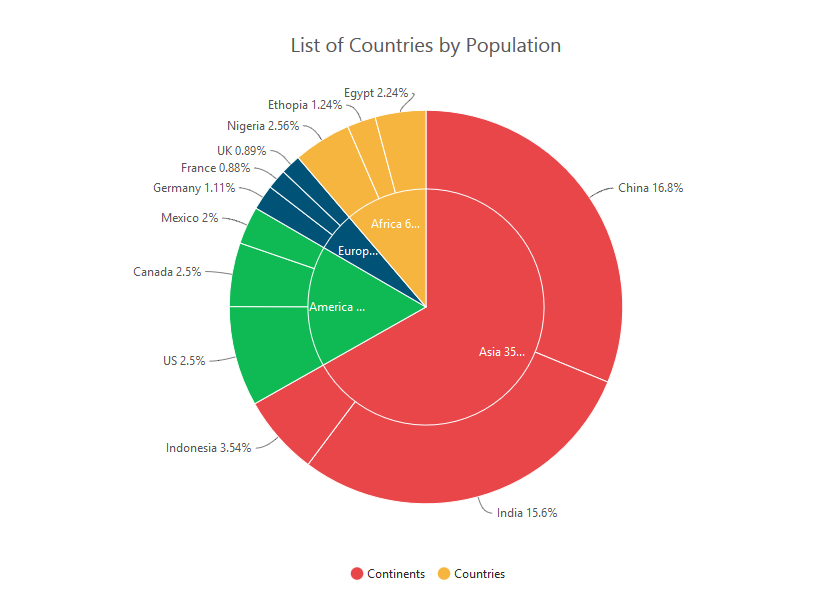Multiple doughnut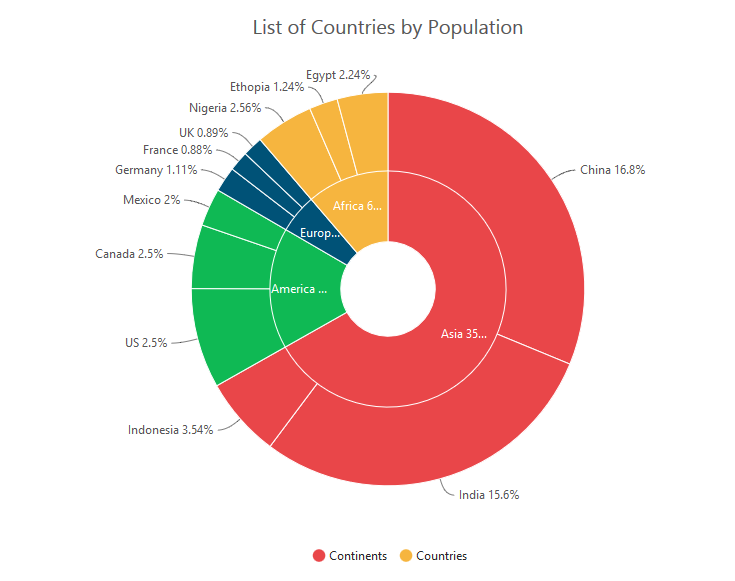### Start and end angles support

In the multiple pie chart, the StartAngle and EndAngle properties are also supported.

Sector of multiple pieSector of multiple doughnut## Pyramid Chart

To create a pyramid chart, specify the series type to Pyramid in the chart series.

• CSHTML
• ``````<ej-chart id="chartContainer">
// ...
<e-chart-series>
<e-series type="Pyramid">
</e-series>
</e-chart-series>
// ...
</ej-chart>``````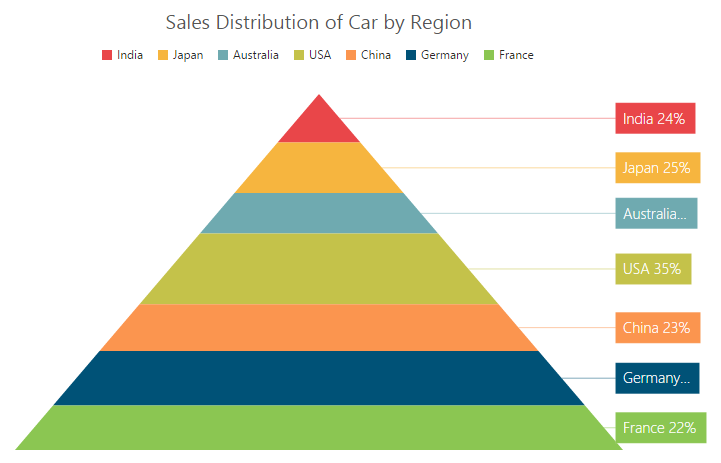### Pyramid mode

Pyramid mode has two types, namely Linear and Surface. The default PyramidMode type is “Linear”.

• CSHTML
• ``````<ej-chart id="chartContainer">
// ...
<e-chart-series>
<e-series pyramid-mode="Surface">
</e-series>
</e-chart-series>
// ...
</ej-chart>``````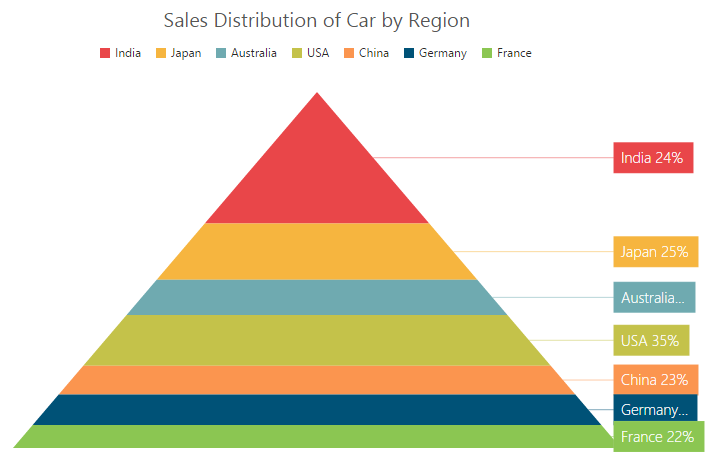### Gap between the segments

You can control the gap between the segments by using the GapRatio property of the series. It ranges from 0 to 1.

• CSHTML
• ``````<ej-chart id="chartContainer">
// ...
<e-chart-series>
<e-series gap-ratio="0.1f">
</e-series>
</e-chart-series>
// ...
</ej-chart>``````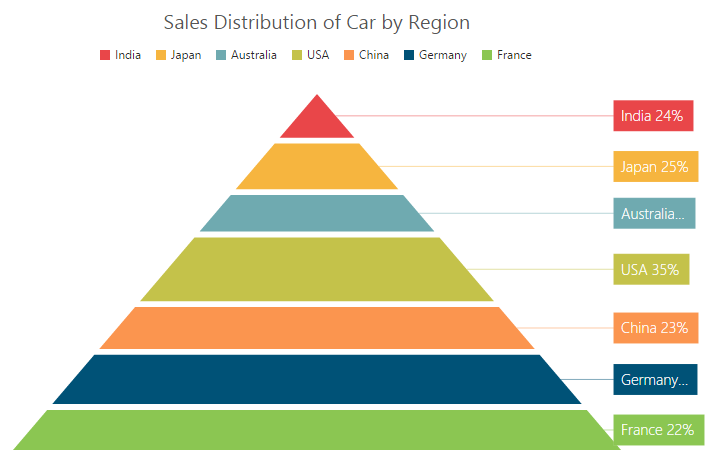### Explode a pyramid segment

You can explode a pyramid segment on the chart load by using the ExplodeIndex property of the series.

• CSHTML
• ``````<ej-chart id="chartContainer">
// ...
<e-chart-series>
<e-series explode-index="4">
</e-series>
</e-chart-series>
// ...
</ej-chart>``````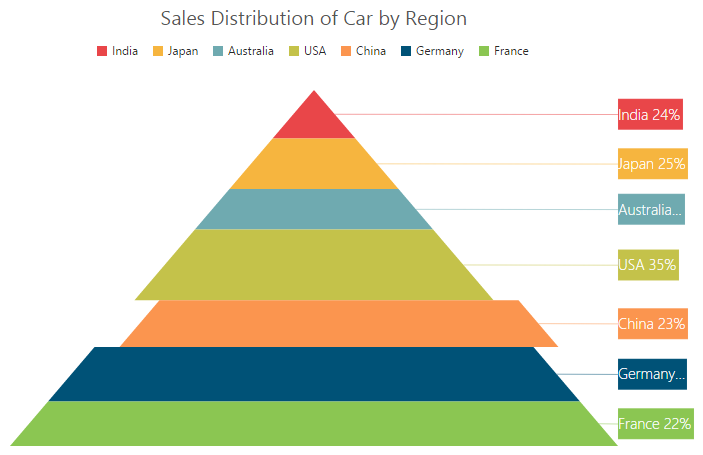## Funnel Chart

You can create a funnel chart by setting the series type to Funnel in the chart series.

• CSHTML
• ``````<ej-chart id="chartContainer">
// ...
<e-chart-series>
<e-series type="Funnel">
</e-series>
</e-chart-series>
// ...
</ej-chart>``````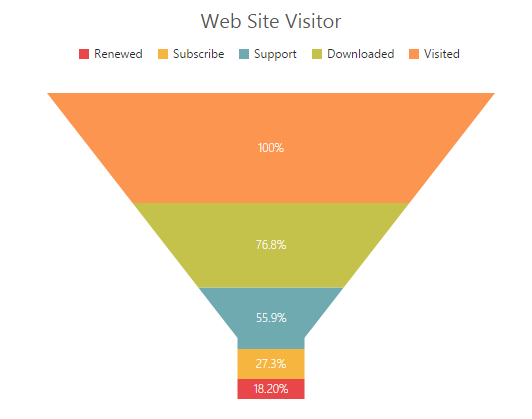### Change the funnel width and height

Funnel segment’s height and width are calculated from the chart size, by default. You can change height and width directly without changing the chart size by using the FunnelHeight and FunnelWidth properties of the series.

• CSHTML
• ``````<ej-chart id="chartContainer">
// ...
<e-chart-series>
<e-series funnel-height="22%" funnel-width="25%">
</e-series>
</e-chart-series>
// ...
</ej-chart>``````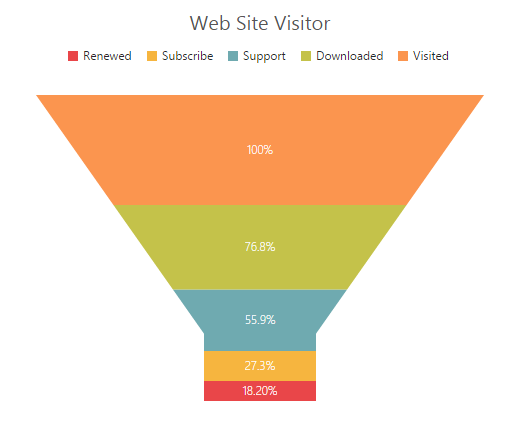### Explode a funnel segment

You can explode a funnel segment on the chart load by using the ExplodeIndex of the series.

• CSHTML
• ``````<ej-chart id="chartContainer">
// ...
<e-chart-series>
<e-series explode-index="3">
</e-series>
</e-chart-series>
// ...
</ej-chart>``````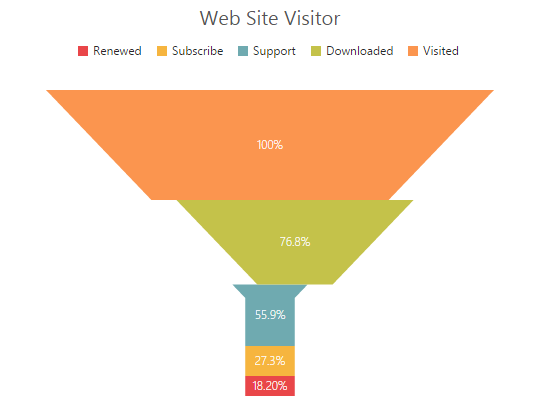## Bubble Chart

To create a Bubble chart, set the series type to Bubble in the chart series. Bubble chart requires 3 fields, namely X, Y, and Size to plot a point. Here, Size is used to specify the size of each bubble segment.

• CSHARP
• ``````// Create dataSource to bubble chart
List<ChartData> data =new List<ChartData>();
ViewBag.ChartData = data;``````
• CSHTML
• ``````<ej-chart id="chartContainer">
// ...
<e-chart-series>
<e-series type="Bubble" x-name="Month" y-name="Sales" size="Profit" datasource="ViewBag.ChartData">
</e-series>
</e-chart-series>
// ...
</ej-chart>``````## Scatter

To create a scatter chart, set the series type to Scatter in the chart series.

• CSHTML
• ``````<ej-chart id="chartContainer">
// ...
<e-chart-series>
<e-series type="Scatter">
</e-series>
</e-chart-series>
// ...
</ej-chart>``````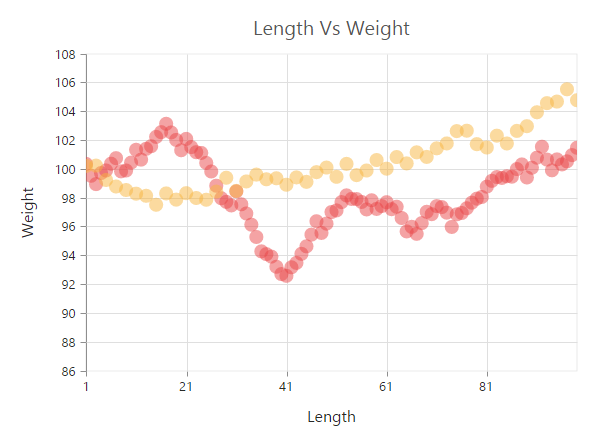### Customize the scatter chart

You can change the scatter size and scatter color by using the Size and Fill properties of the series marker, respectively.

• CSHTML
• ``````<ej-chart id="chartContainer">
// ...
<e-chart-series>
<e-series><e-marker><e-Size height="15" width="15"></e-Size></e-marker>
</e-series>
</e-chart-series>
// ...
</ej-chart>``````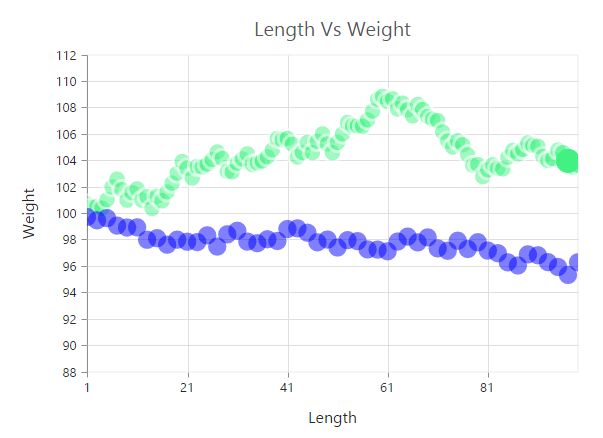## HiLoOpenClose Chart

To create a HiLo open close chart, set the series type to HiloOpenClose in the chart series. HiLo open close chart requires 5 fields, namely X, High, Low, Open, and Close to plot a segment.

• CSHARP
• ``````// Create dataSource to chart
List<ChartData> data =new List<ChartData>();
data.Add(new ChartData("Feb", 28, 15, 18, 27));
data.Add(new ChartData("Mar", 54, 35, 38, 49));
data.Add(new ChartData("Apr", 52, 21, 35, 29));
ViewBag.ChartData = data;``````
• CSHTML
• ``````<ej-chart id="chartContainer">
// ...
<e-chart-series>
<e-series type="HiloOpenClose" x-name="Month" high="High" low="Low" open="Open" close="Close" datasource="ViewBag.ChartData">
</e-series>
</e-chart-series>
// ...
</ej-chart>``````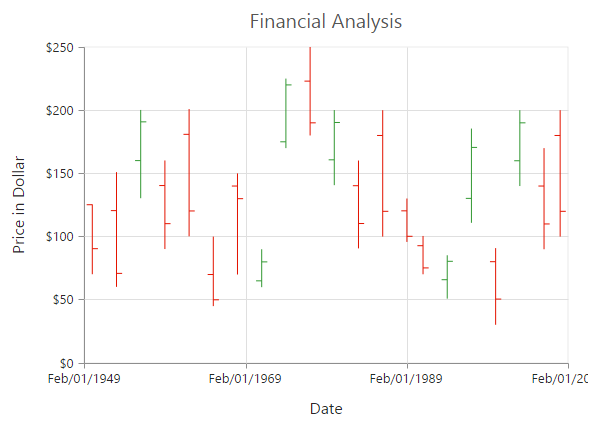### DrawMode

You can change the HiLo open close chart’s DrawMode option to Open, Close, or Both. The default value of DrawMode is Both.

• CSHTML
• ``````<ej-chart id="chartContainer">
// ...
<e-chart-series>
<e-series draw-mode="Open">
</e-series>
</e-chart-series>
// ...
</ej-chart>``````### Bull and bear Color

BullFillColor of HiLo open close chart is used to specify a fill color for the segments that indicates an increase in stock price in the measured time interval, and BearFillColor is used to specify a fill color for the segments that indicates a decrease in the stock price in the measured time interval.

• CSHTML
• ``````<ej-chart id="chartContainer">
// ...
<e-chart-series>
<e-series bear-fill-color="#FF6600" bull-fill-color="#336600">
</e-series>
</e-chart-series>
// ...
</ej-chart>``````## Candle

You can create a Candle chart by specifying the series type to Candle in the chart series. Candle chart requires 5 fields, namely X, High, Low, Open, and Close to plot a segment.

• CSHARP
• ``````// Create dataSource to chart
List<ChartData> data =new List<ChartData>();
data.Add(new ChartData("Feb", 28, 15, 18, 27));
data.Add(new ChartData("Mar", 54, 35, 38, 49));
data.Add(new ChartData("Apr", 52, 21, 35, 29));
ViewBag.ChartData = data;``````
• CSHTML
• ``````<ej-chart id="chartContainer">
// ...
<e-chart-series>
<e-series type="Candle" x-axis-name="Month" high="High" low="Low" open="Open" close="Close" datasource="ViewBag.ChartData">
</e-series>
</e-chart-series>
// ...
</ej-chart>``````### Bull and Bear Color

BullFillColor of candle chart is used to specify a fill color for the segments that indicates an increase in the stock price in the measured time interval and, BearFillColor is used to specify a fill color for the segments that indicates a decrease in the stock price in the measured time interval.

• CSHTML
• ``````<ej-chart id="chartContainer">
// ...
<e-chart-series>
<e-series bear-fill-color="#FF6600" bull-fill-color="#336600">
</e-series>
</e-chart-series>
// ...
</ej-chart>``````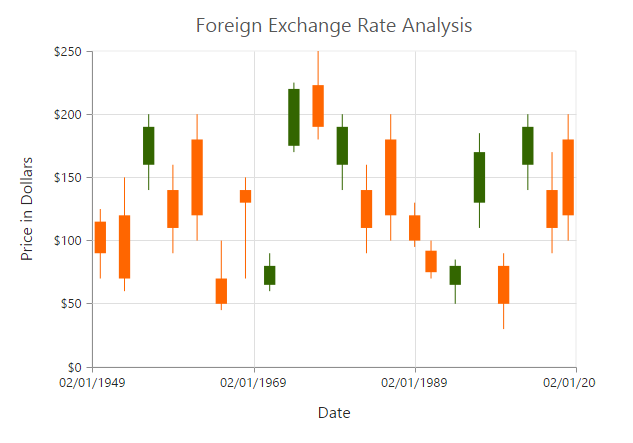## HiLo

HiLo chart is created by setting the series type to Hilo in the chart series. HiLo chart requires 3 fields, namely X, High, and Low to plot a segment.

• CSHARP
• ``````// Create dataSource to chart
List<ChartData> data =new List<ChartData>();
ViewBag.ChartData = data;``````
• CSHTML
• ``````<ej-chart id="chartContainer">
// ...
<e-chart-series>
<e-series type="Hilo" x-axis-name="Month" high="High" low="Low">
</e-series>
</e-chart-series>
// ...
</ej-chart>``````## Polar

Polar chart is created by setting the series type to Polar in the chart series.

• CSHTML
• ``````<ej-chart id="chartContainer">
// ...
<e-chart-series>
<e-series type="Polar">
</e-series>
</e-chart-series>
// ...
</ej-chart>``````### DrawType

Polar DrawType property is used to change the series plotting type to Line, scatter, rangeColumn, stackingArea, spline, Column or Area. The default value of DrawType is Line.

• CSHTML
• ``````<ej-chart id="chartContainer">
// ...
<e-chart-series>
<e-series draw-type="Column">
</e-series>
</e-chart-series>
// ...
</ej-chart>``````### Stack columns in polar chart

By using the IsStacking property, you can specify whether the column has to be stacked when the draw type is column. Its default value is false.

• CSHTML
• ``````<ej-chart id="chartContainer">
// ...
<e-chart-series>
<e-series is-stacking="true">
</e-series>
</e-chart-series>
// ...
</ej-chart>``````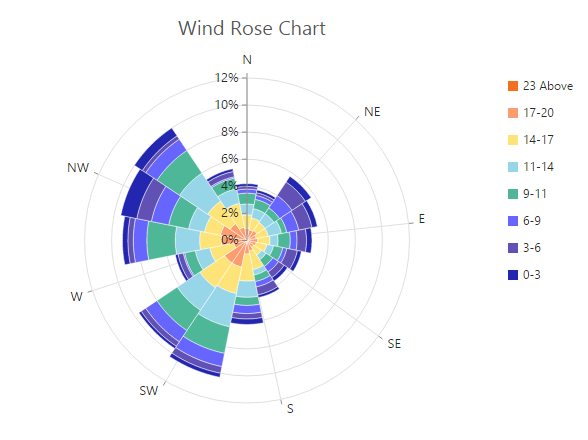To create a radar chart, specify the series type to Radar in the chart series.

• CSHTML
• ``````<ej-chart id="chartContainer">
// ...
<e-chart-series>
</e-series>
</e-chart-series>
// ...
</ej-chart>``````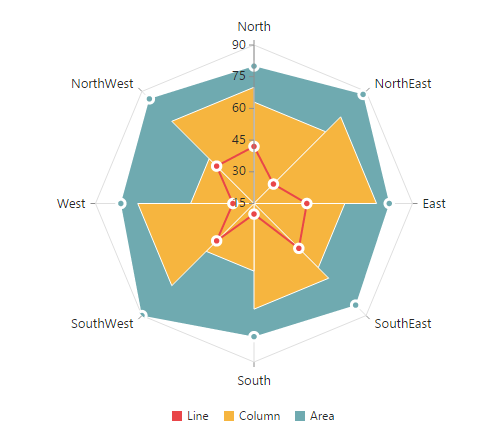### DrawType

Radar DrawType property is used to change the series plotting type to Line, scatter, rangeColumn, stackingArea, spline, Column or Area. The default value of DrawType is Line.

• CSHTML
• ``````<ej-chart id="chartContainer">
// ...
<e-chart-series>
<e-series draw-type="Column">
</e-series>
</e-chart-series>
// ...
</ej-chart>``````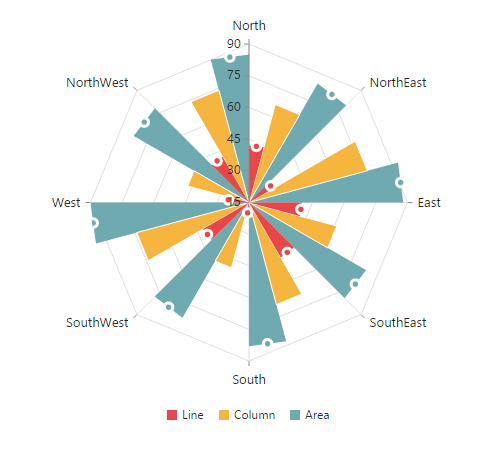### Stack columns in Radar chart

By using the IsStacking property, you can specify whether the column has to be stacked when the draw type is set to Column. Its default value is false.

• CSHTML
• ``````<ej-chart id="chartContainer">
// ...
<e-chart-series>
<e-series is-stacking="true">
</e-series>
</e-chart-series>
// ...
</ej-chart>``````## Waterfall Chart

For rendering a waterfall chart, set series type to Waterfall in the chart series. To change the color of the waterfall series segment, use Fill option of series, and use PositiveFill property to differentiate the positive segments.

NOTE

The inline property of the Series.PositiveFill has the first priority, and this overrides the Series.Fill property.

• CSHTML
• ``````<ej-chart id="chartContainer">
// ...
<e-chart-series>
<e-series type="Waterfall" fill="#C64E4A" positive-fill="#93C952">
</e-series>
</e-chart-series>
// ...
</ej-chart>``````ShowIntermediateSum

To display the summary of values from the last intermediate point of the waterfall series, set ShowIntermediateSum property to true in the specific point.

• CSHTML
• ``````<ej-chart id="chartContainer">
// ...
<e-chart-series>
<e-series>
<e-points>
<e-point show-intermediate-sum="true" x="Intermediate sum"></e-point>
</e-points>
</e-series>
</e-chart-series>
// ...
</ej-chart>``````

ShowTotalSum

The sum of all previous points in the waterfall series is displayed by enabling the ShowTotalSum property for a specific point.

• CSHTML
• ``````<ej-chart id="chartContainer">
// ...
<e-chart-series>
<e-series>
<e-points>
<e-point show-total-sum="true" x="Total sum"></e-point>
</e-points>
</e-series>
</e-chart-series>
// ...
</ej-chart>``````

### ConnectorLine

To customize the connector line color, width, opacity, and dashArray of the waterfall series, use ConnectorLine option of series.

• CSHTML
• ``````<ej-chart id="chartContainer">
// ...
<e-chart-series>
<e-series width="3" opacity="1" dash-array="2,3">
<e-connector-line color="#333000"></e-connector-line>
</e-series>
</e-chart-series>
// ...
</ej-chart>``````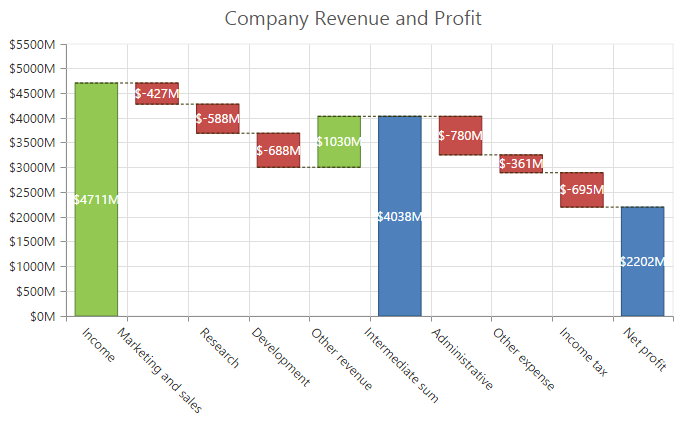## Error Bar Chart

Chart can generate error bar for Cartesian type series, such as line, column, bar, scatter, area, candle, HiLo, etc. To render the error bar for the series, set Visibility as visible to ErrorBar in the series.

• CSHTML
• ``````<ej-chart id="chartContainer">
// ...
<e-chart-series>
<e-series>
<e-error-bar visibility="true"></e-error-bar>
</e-series>
</e-chart-series>
// ...
</ej-chart>``````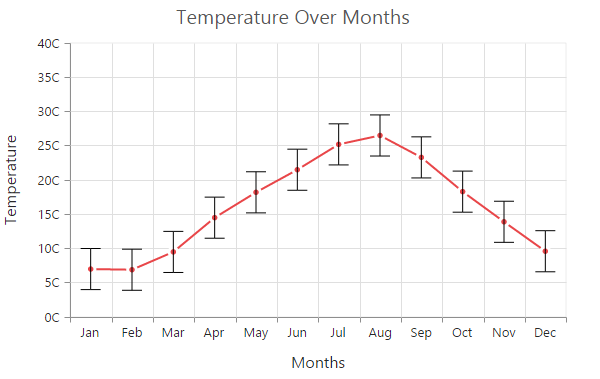### Changing error bar type

You can change the error bar rendering type by using Type (like FixedValue, Percentage, StandardDeviation, StandardError and Custom) option of error bar. To change the error bar line length, use VerticalErrorValue property.

• CSHTML
• ``````<ej-chart id="chartContainer">
// ...
<e-chart-series>
<e-series>
<e-error-bar type="FixedValue" vertical-error-value="3"></e-error-bar>
</e-series>
</e-chart-series>
// ...
</ej-chart>``````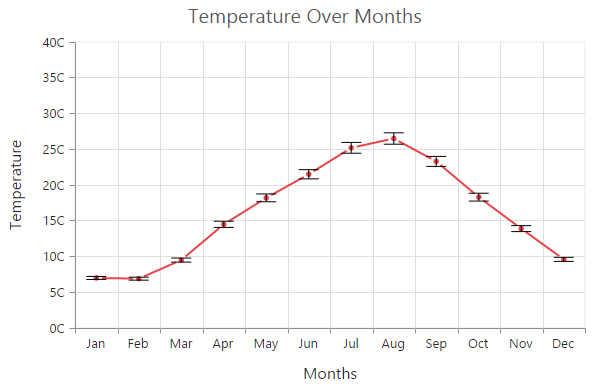#### Customizing error bar type

To customize the error bar type, set error bar type to Custom, and then change the horizontal/vertical positive and negative values of error bar.

• CSHTML
• ``````<ej-chart id="chartContainer">
// ...
<e-chart-series>
<e-series>
<e-error-bar type="Custom" vertical-positive-error-value="5" vertical-negative-error-value="5" horizontal-positive-error-value="1" horizontal-negative-error-value="1"></e-error-bar>
</e-series>
</e-chart-series>
// ...
</ej-chart>``````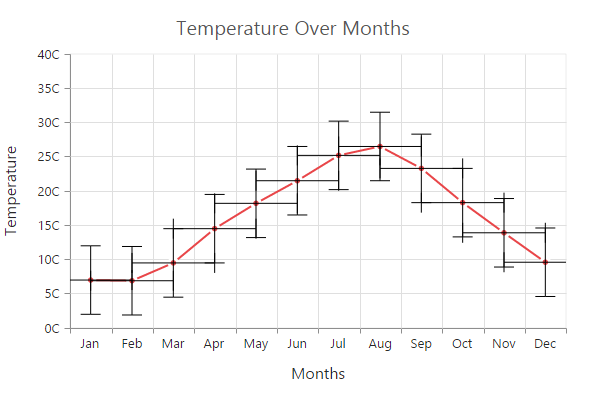### Changing error bar mode

Error bar mode is used to define whether the error bar line has to be drawn Horizontally, Vertically, or in Both side. To change the error bar mode, use ErrorBar.Mode option.

• CSHTML
• ``````<ej-chart id="chartContainer">
// ...
<e-chart-series>
<e-series>
<e-error-bar type="FixedValue" mode="Vertical"></e-error-bar>
</e-series>
</e-chart-series>
// ...
</ej-chart>``````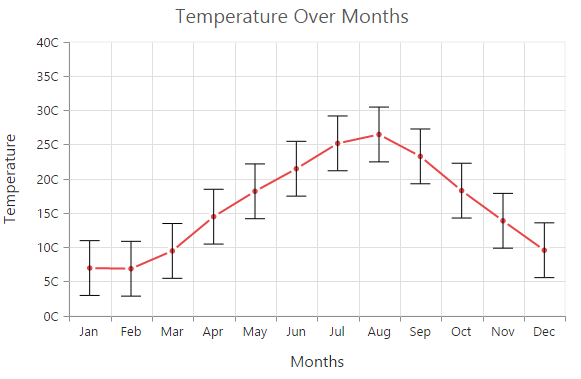### Changing error bar direction

You can change the error bar direction to plus, minus, or both side by using ErrorBar.Directions option.

• CSHTML
• ``````<ej-chart id="chartContainer">
// ...
<e-chart-series>
<e-series>
<e-error-bar type="FixedValue" mode="Vertical" direction="Minus"></e-error-bar>
</e-series>
</e-chart-series>
// ...
</ej-chart>``````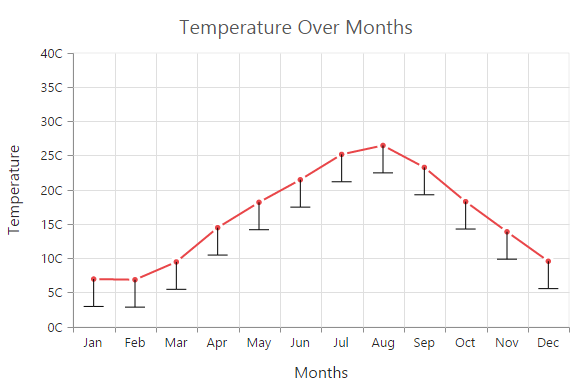### Customizing error bar cap

To customize the visibility, length, width, and fill color of error bar cap, use Cap option in the Series.ErrorBar.

• CSHTML
• ``````<ej-chart id="chartContainer">
// ...
<e-chart-series>
<e-series>
<e-error-bar><e-cap visible="true" cap-length="20" cap-width="1" cap-fill="#000000"></e-cap></e-error-bar>
</e-series>
</e-chart-series>
// ...
</ej-chart>``````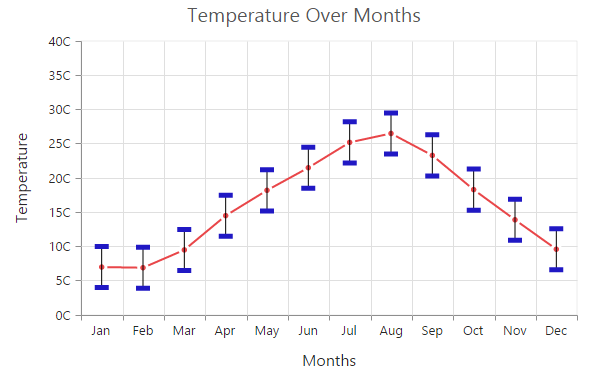## Box and Whisker Chart

To render a box and whisker chart, set the series type to boxAndWhisker. This chart requires 2 fields, namely x and y to plot a segment. The field y requires n number of data, or it should contains minimum of five values to plot a segment.### BoxPlotMode

You can change the rendering mode of the box and whisker series by using the BoxPlotMode property. The default value of BoxPlotMode is exclusive. The other boxPlotModes available are `inclusive` and `normal`.

• CSHTML
• ``````<ej-chart id="chartContainer">
// ...
<e-chart-series>
<e-series box-plot-mode="Inclusive">
</e-series>
</e-chart-series>
// ...
</ej-chart>``````

### Customize the outlier

Outlier symbol’s width and height can be customized by using outlierSettings through outlierSettings property. By default outlier symbol is displayed as circle with a height and width of 6 pixels.

• CSHTML
• ``````<ej-chart id="chartContainer">
// ...
<e-size height="10" width="10"></e-size>
<e-chart-series>
<e-series>
<e-outlier-settings shape="Triangle"></e-outlier-settings>
</e-series>
</e-chart-series>
// ...
</ej-chart>``````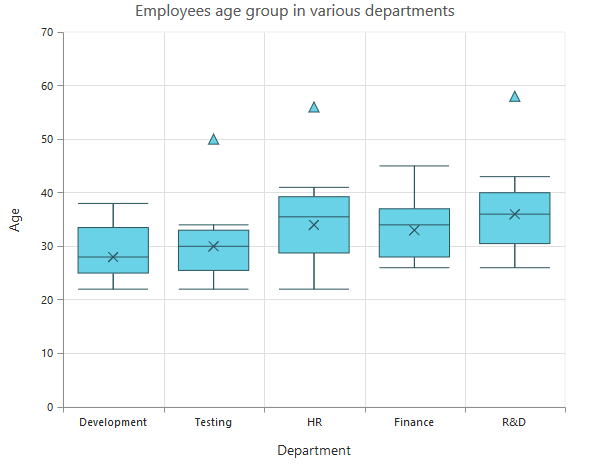## Pie of Pie Chart

To render the pie of pie chart, set the series type to pieofpie. Pie of pie chart is used for displaying the data of a pie slice as another pie chart. The values in the second pie is displayed based on the splitMode property.

• CSHTML
• ``````<ej-chart id="chartContainer">
// ...
<e-chart-series>
<e-series type="pieofpie">
</e-series>
</e-chart-series>
// ...
</ej-chart>``````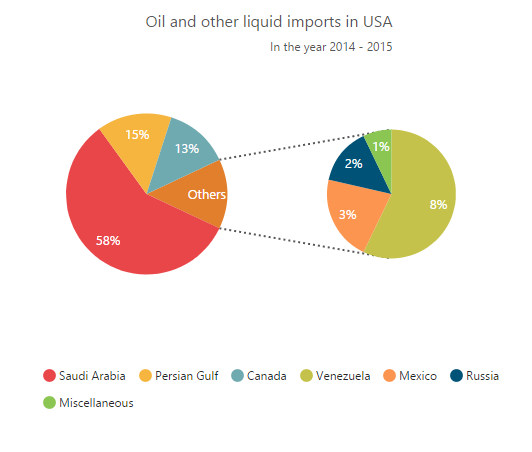### Split mode and split value

The points to be displayed in the second pie is decided based on the `SplitMode` property. This property takes the following values:

• Position – Have to split the data points based on its position.
• Value – Have to split the data points based on its Y value
• Percentage – Have to split the points based on the percentage value.
• Indexes – The data points with the specified indexes are split separately.

By default, the splitMode is set to Value.

• CSHTML
• ``````<ej-chart id="chartContainer">
// ...
<e-chart-series>
<e-series split-mode="Position" split-value="3">
</e-series>
</e-chart-series>
// ...
</ej-chart>``````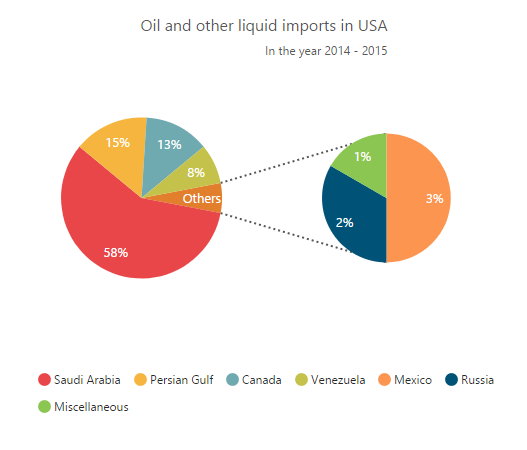### Changing pie Of pie size

The size of the second pie can be customized by using the PieOfPieCoefficient property. The default value of pieOfPieCoefficient is 0.6. Its value ranges from 0 to 1.

• CSHTML
• ``````<ej-chart id="chartContainer">
// ...
<e-chart-series>
<e-series pie-of-pie-coefficient="1">
</e-series>
</e-chart-series>
// ...
</ej-chart>``````

The following screenshot represents the pie of pie series with pieOfPieCoefficient as 1.#### Customizing the Gap

The distance between the two pies in the pie of pie chart can be controlled by using the GapWidth property. Its default value is 50.

• CSHTML
• ``````<ej-chart id="chartContainer">
// ...
<e-chart-series>
<e-series gap-width="150">
</e-series>
</e-chart-series>
// ...
</ej-chart>``````